Author Message
Eric M. Jones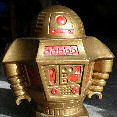Joined: 10 Jan 2006
Posts: 561
Location: MassachusettsPosted: Fri Oct 17, 2014 10:06 am    Post subject: Crankcase ferrous particle detectors?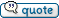```Who makes affordable crankcase ferrous particle detectors? Eric```

 - The Matronics AeroElectric-List Email Forum - Use the List Feature Navigator to browse the many List utilities available such as the Email Subscriptions page, Archive Search & Download, 7-Day Browse, Chat, FAQ, Photoshare, and much more:http://www.matronics.com/Navigator?AeroElectric-List

_________________
Eric M. Jones
www.PerihelionDesign.com
113 Brentwood Drive
Southbridge, MA 01550
(508) 764-2072
emjones(at)charter.net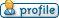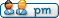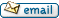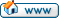Bill Allen

Joined: 24 Aug 2009
Posts: 40
Location: UKPosted: Fri Oct 17, 2014 10:37 am    Post subject: Crankcase ferrous particle detectors?```Hi Eric, you wrote <<Who makes affordable crankcase ferrous particle detectors?>> I may have misunderstood this to mean a "chip detector" Â which I have fitted to my Lycoming 0-320 engined aircraft.Â  I sourced mine from a breaker - there are plenty of crashed Robinson R22s out there, and all have chip/particle detectors in their gearboxes. I don't know the original source of these when new, but they're affordable from the breaker. my 10c FWIW Bill AllenLongEz160 N99BA FD51 CZ4 G-BYLZ EGBJ On 17 October 2014 20:06, Eric M. Jones <emjones(at)charter.net (emjones(at)charter.net)> wrote: Quote: --> AeroElectric-List message posted by: "Eric M. Jones" <emjones(at)charter.net (emjones(at)charter.net)> Who makes affordable crankcase ferrous particle detectors? Eric -------- Eric M. Jones www.PerihelionDesign.com 113 Brentwood Drive Southbridge, MA 01550 (508) 764-2072 emjones(at)charter.net Read this topic online here: http://forums.matronics.com/viewtopic.php?p=431984#431984 =========== - Electric-List" target="_blank">http://www.matronics.com/Navigator?AeroElectric-List =========== FORUMS - _blank">http://forums.matronics.com =========== b Site - Â  Â  Â  Â  Â  -Matt Dralle, List Admin. target="_blank">http://www.matronics.com/contribution =========== -- Bill AllenLongEz160 N99BA FD51 CZ4 G-BYLZ EGBJ [quote][b]```

 - The Matronics AeroElectric-List Email Forum - Use the List Feature Navigator to browse the many List utilities available such as the Email Subscriptions page, Archive Search & Download, 7-Day Browse, Chat, FAQ, Photoshare, and much more:http://www.matronics.com/Navigator?AeroElectric-Listedpav8r(at)yahoo.com
GuestPosted: Fri Oct 17, 2014 11:54 am    Post subject: Crankcase ferrous particle detectors?```What's the output from one of these sensors, and what are you monitoring/displaying it with? Eric On Oct 17, 2014, at 11:36 AM, Bill Allen <billallensworld(at)gmail.com (billallensworld(at)gmail.com)> wrote: [quote]I may have misunderstood this to mean a "chip detector" which I have fitted to my Lycoming 0-320 engined aircraft. I sourced mine from a breaker - there are plenty of crashed Robinson R22s out there, and all have chip/particle detectors in their gearboxes. I don't know the original source of these when new, but they're affordable from the breaker. my 10c FWIW Bill AllenLongEz160 N99BA FD51 CZ4 G-BYLZ EGBJ [b]```

 - The Matronics AeroElectric-List Email Forum - Use the List Feature Navigator to browse the many List utilities available such as the Email Subscriptions page, Archive Search & Download, 7-Day Browse, Chat, FAQ, Photoshare, and much more:http://www.matronics.com/Navigator?AeroElectric-Listedpav8r(at)yahoo.com
GuestPosted: Fri Oct 17, 2014 12:12 pm    Post subject: Crankcase ferrous particle detectors?```They're called wreckers in the auto business. They break up junk aircraft and sell off parts, then recycle the hull. Eric On Oct 17, 2014, at 12:29 PM, <rnjcurtis(at)charter.net (rnjcurtis(at)charter.net)> wrote: at the risk of sounding stupid, may I ask, who is "the breaker"? Quote: Quote: [quote][b]```

 - The Matronics AeroElectric-List Email Forum - Use the List Feature Navigator to browse the many List utilities available such as the Email Subscriptions page, Archive Search & Download, 7-Day Browse, Chat, FAQ, Photoshare, and much more:http://www.matronics.com/Navigator?AeroElectric-Listjmjones2000(at)mindspring
GuestPosted: Fri Oct 17, 2014 12:14 pm    Post subject: Crankcase ferrous particle detectors?```Most of the chip detectors are in the shape of a spark plug, but smaller. They have two magnetic posts that are also electrical contacts. When enough ferrous metal collects on the magnetic co facts, it closes a circuit. You can wire it to illuminate a light on the panel or to display on a dynon engine monitor. Other monitors may display the condition of a contact as well. Hope this helps. Justin On Oct 17, 2014, at 13:50, Eric Page <edpav8r(at)yahoo.com (edpav8r(at)yahoo.com)> wrote: [quote]What's the output from one of these sensors, and what are you monitoring/displaying it with? Eric On Oct 17, 2014, at 11:36 AM, Bill Allen <billallensworld(at)gmail.com (billallensworld(at)gmail.com)> wrote: Quote: I may have misunderstood this to mean a "chip detector" which I have fitted to my Lycoming 0-320 engined aircraft. I sourced mine from a breaker - there are plenty of crashed Robinson R22s out there, and all have chip/particle detectors in their gearboxes. I don't know the original source of these when new, but they're affordable from the breaker. my 10c FWIW Bill AllenLongEz160 N99BA FD51 CZ4 G-BYLZ EGBJ D=3D=3D=3D=3D=3D=3D=3D=3D=3D=3D=3D=3D=3D=3D=3D=3D=3D=3D=3D=3D=3D=3D=3D=3D=3D=3D=3D=3D=3D=3D=3D=3D=3D=3D=3D=3D=3D=3D=3D=3D=3D=3D=3D=3D=3D lectric-List"">http://www.matronics.com/Navigator?AeroElectric-List D=3D=3D=3D=3D=3D=3D=3D=3D=3D=3D=3D=3D=3D=3D=3D=3D=3D=3D=3D=3D=3D=3D=3D=3D=3D=3D=3D=3D=3D=3D=3D=3D=3D=3D=3D=3D=3D=3D=3D=3D=3D=3D=3D=3D=3D //forums.matronics.com D=3D=3D=3D=3D=3D=3D=3D=3D=3D=3D=3D=3D=3D=3D=3D=3D=3D=3D=3D=3D=3D=3D=3D=3D=3D=3D=3D=3D=3D=3D=3D=3D=3D=3D=3D=3D=3D=3D=3D=3D=3D=3D=3D=3D=3D ot;">http://www.matronics.com/contribution D=3D=3D=3D=3D=3D=3D=3D=3D=3D=3D=3D=3D=3D=3D=3D=3D=3D=3D=3D=3D=3D=3D=3D=3D=3D=3D=3D=3D=3D=3D=3D=3D=3D=3D=3D=3D=3D=3D=3D=3D=3D=3D=3D=3D=3D [b]```

 - The Matronics AeroElectric-List Email Forum - Use the List Feature Navigator to browse the many List utilities available such as the Email Subscriptions page, Archive Search & Download, 7-Day Browse, Chat, FAQ, Photoshare, and much more:http://www.matronics.com/Navigator?AeroElectric-Listjmjones2000(at)mindspring
GuestPosted: Fri Oct 17, 2014 12:30 pm    Post subject: Crankcase ferrous particle detectors?```I meant to say contacts. Not co facts. On Oct 17, 2014, at 14:13, Justin Jones <jmjones2000(at)mindspring.com (jmjones2000(at)mindspring.com)> wrote: [quote]Most of the chip detectors are in the shape of a spark plug, but smaller. They have two magnetic posts that are also electrical contacts. When enough ferrous metal collects on the magnetic co facts, it closes a circuit. You can wire it to illuminate a light on the panel or to display on a dynon engine monitor. Other monitors may display the condition of a contact as well. Hope this helps. Justin On Oct 17, 2014, at 13:50, Eric Page <edpav8r(at)yahoo.com (edpav8r(at)yahoo.com)> wrote: Quote: What's the output from one of these sensors, and what are you monitoring/displaying it with? Eric On Oct 17, 2014, at 11:36 AM, Bill Allen <billallensworld(at)gmail.com (billallensworld(at)gmail.com)> wrote: Quote: I may have misunderstood this to mean a "chip detector" which I have fitted to my Lycoming 0-320 engined aircraft. I sourced mine from a breaker - there are plenty of crashed Robinson R22s out there, and all have chip/particle detectors in their gearboxes. I don't know the original source of these when new, but they're affordable from the breaker. my 10c FWIW Bill AllenLongEz160 N99BA FD51 CZ4 G-BYLZ EGBJ D=3D=3D=3D=3D=3D=3D=3D=3D=3D=3D=3D=3D=3D=3D=3D=3D=3D=3D=3D=3D=3D=3D=3D=3D=3D=3D=3D=3D=3D=3D=3D=3D=3D=3D=3D=3D=3D=3D=3D=3D=3D=3D=3D=3D=3D lectric-List"">http://www.matronics.com/Navigator?AeroElectric-List D=3D=3D=3D=3D=3D=3D=3D=3D=3D=3D=3D=3D=3D=3D=3D=3D=3D=3D=3D=3D=3D=3D=3D=3D=3D=3D=3D=3D=3D=3D=3D=3D=3D=3D=3D=3D=3D=3D=3D=3D=3D=3D=3D=3D=3D //forums.matronics.com D=3D=3D=3D=3D=3D=3D=3D=3D=3D=3D=3D=3D=3D=3D=3D=3D=3D=3D=3D=3D=3D=3D=3D=3D=3D=3D=3D=3D=3D=3D=3D=3D=3D=3D=3D=3D=3D=3D=3D=3D=3D=3D=3D=3D=3D ot;">http://www.matronics.com/contribution D=3D=3D=3D=3D=3D=3D=3D=3D=3D=3D=3D=3D=3D=3D=3D=3D=3D=3D=3D=3D=3D=3D=3D=3D=3D=3D=3D=3D=3D=3D=3D=3D=3D=3D=3D=3D=3D=3D=3D=3D=3D=3D=3D=3D=3D D=3D=3D=3D=3D=3D=3D=3D=3D=3D=3D=3D=3D=3D=3D=3D=3D=3D=3D=3D=3D=3D=3D=3D=3D=3D=3D=3D=3D=3D=3D=3D=3D=3D=3D=3D=3D=3D=3D=3D=3D=3D=3D=3D=3D=3D lectric-List"">http://www.matronics.com/Navigator?AeroElectric-List D=3D=3D=3D=3D=3D=3D=3D=3D=3D=3D=3D=3D=3D=3D=3D=3D=3D=3D=3D=3D=3D=3D=3D=3D=3D=3D=3D=3D=3D=3D=3D=3D=3D=3D=3D=3D=3D=3D=3D=3D=3D=3D=3D=3D=3D //forums.matronics.com D=3D=3D=3D=3D=3D=3D=3D=3D=3D=3D=3D=3D=3D=3D=3D=3D=3D=3D=3D=3D=3D=3D=3D=3D=3D=3D=3D=3D=3D=3D=3D=3D=3D=3D=3D=3D=3D=3D=3D=3D=3D=3D=3D=3D=3D ot;">http://www.matronics.com/contribution D=3D=3D=3D=3D=3D=3D=3D=3D=3D=3D=3D=3D=3D=3D=3D=3D=3D=3D=3D=3D=3D=3D=3D=3D=3D=3D=3D=3D=3D=3D=3D=3D=3D=3D=3D=3D=3D=3D=3D=3D=3D=3D=3D=3D=3D [b]```

 - The Matronics AeroElectric-List Email Forum - Use the List Feature Navigator to browse the many List utilities available such as the Email Subscriptions page, Archive Search & Download, 7-Day Browse, Chat, FAQ, Photoshare, and much more:http://www.matronics.com/Navigator?AeroElectric-ListBob McC

Joined: 09 Jan 2006
Posts: 258
Location: Toronto, ONPosted: Fri Oct 17, 2014 12:41 pm    Post subject: Crankcase ferrous particle detectors?```"The breakers" is the English term for what in the US is commonly referred to as a "wrecking yard" or "junk yard". In this context it would also mean "aircraft salvage yard". Bob McC From: rnjcurtis(at)charter.net To: aeroelectric-list(at)matronics.com Subject: Re: AeroElectric-List: Crankcase ferrous particle detectors? Date: Fri, 17 Oct 2014 19:29:24 +0000 .ExternalClass p.ecxMsoListParagraph, .ExternalClass li.ecxMsoListParagraph, .ExternalClass div.ecxMsoListParagraph { } .ExternalClass p.ecxMsoNormal, .ExternalClass li.ecxMsoNormal, .ExternalClass div.ecxMsoNormal { } .ExternalClass p.ecxMsoListParagraphCxSpFirst, .ExternalClass li.ecxMsoListParagraphCxSpFirst, .ExternalClass div.ecxMsoListParagraphCxSpFirst, .ExternalClass p.ecxMsoListParagraphCxSpMiddle, .ExternalClass li.ecxMsoListParagraphCxSpMiddle, .ExternalClass div.ecxMsoListParagraphCxSpMiddle, .ExternalClass p.ecxMsoListParagraphCxSpLast, .ExternalClass li.ecxMsoListParagraphCxSpLast, .ExternalClass div.ecxMsoListParagraphCxSpLast { line-height:115%; } I don't know the original source of these when new, but they're affordable from the breaker. Quote: at the risk of sounding stupid, may I ask, who is âthe breakerâ? Roger )ï¿½ï¿½ß¢{lï¿½7ï¿½rï¿½hï¿½M4ï¿½MiÇï¿½ï¿½ï¿½zï¿½ï¿½ï¿½ï¿½.ï¿½'ï¿½Nï¿½zï¿½ï¿½ï¿½-ï¿½' ï¿½ï¿½Dï¿½ï¿½ï¿½ï¿½ï¿½Kï¿½ï¿½jï¿½ï¿½',.+-æ­ºï¿½ï¿½5ï¿½â«hï¿½ï¿½ï¿½ï¿½,zï¿½^ï¿½ï¿½ï¿½.+-ï¿½Ø¥ï¿½Øï¿½Ëï¿½ï¿½ ï¿½ï¿½Tï¿½ï¿½nï¿½+ï¿½ï¿½bï¿½p+rï¿½y'ï¿½ï¿½ï¿½Cï¿½ å¡§{ ï¿½ï¿½ï¿½ï¿½,x(Zï¿½P>-ï¿½ï¿½Zï¿½ï¿½vkï¿½ï¿½kï¿½ï¿½j+yï¿½kyï¿½mï¿½ï¿½ï¿½ï¿½ &jï¿½ï¿½',rï¿½ï¿½5ï¿½â«hï¿½ï¿½ï¿½I^rï¿½ï¿½pï¿½ï¿½ï¿½mï¿½ï¿½ï¿½ï¿½ ï¿½ï¿½ï¿½'ï¿½ï¿½ï¿½oï¿½jï¿½ï¿½jï¿½+ï¿½ï¿½Wï¿½ï¿½ï¿½ï¿½.+-ï¿½ï¿½Mï¿½ \$ï¿½NECIï¿½ï¿½ï¿½ï¿½ï¿½ï¿½ï¿½'ï¿½ï¿½ï¿½j[(jï¿½ï¿½ï¿½ï¿½ï¿½zï¿½ï¿½ï¿½ï¿½yï¿½hï¿½ï¿½jï¿½~mï¿½ï¿½ß¢ï¿½ï¿½ï¿½fï¿½ï¿½ï¿½ï¿½rï¿½(ï¿½mï¿½ï¿½ß¢ï¿½ï¿½ï¿½fï¿½ï¿½ï¿½ï¿½rï¿½(ï¿½ï¿½ï¿½ï¿½ï¿½Bï¿½{kï¿½ï¿½ï¿½ï¿½ï¿½ï¿½yï¿½ï¿½ï¿½ï¿½ï¿½jy2ï¿½ï¿½ï¿½*.ï¿½ï¿½zï¿½.ï¿½Ë©ï¿½ï¿½ï¿½1ï¿½mï¿½ï¿½ï¿½ï¿½ï¿½ï¿½ï¿½)Úï¿½ï¿½ï¿½ï¿½iï¿½ï¿½0ï¿½fï¿½ï¿½ï¿½ï¿½rï¿½(ï¿½ï¿½(ï¿½ï¿½ï¿½nï¿½bï¿½xmï¿½ï¿½ï¿½ï¿½ &jï¿½ï¿½',rï¿½ï¿½rï¿½ï¿½&ï¿½*'ï¿½ï¿½ï¿½ï¿½ï¿½'ï¿½ï¿½k{ï¿½ï¿½w/ï¿½i```

 - The Matronics AeroElectric-List Email Forum - Use the List Feature Navigator to browse the many List utilities available such as the Email Subscriptions page, Archive Search & Download, 7-Day Browse, Chat, FAQ, Photoshare, and much more:http://www.matronics.com/Navigator?AeroElectric-List

_________________
Bob McC
Falco #908
(just starting)neal.george(at)gmail.com
GuestPosted: Fri Oct 17, 2014 8:44 pm    Post subject: Crankcase ferrous particle detectors?```Eric - Continental offers a number of sump plugs with integral magnets... Neal George Sent from my iPhone Quote: On Oct 17, 2014, at 1:06 PM, "Eric M. Jones" <emjones(at)charter.net> wrote: Who makes affordable crankcase ferrous particle detectors? Eric -------- Eric M. Jones www.PerihelionDesign.com 113 Brentwood Drive Southbridge, MA 01550 (508) 764-2072 emjones(at)charter.net Read this topic online here: http://forums.matronics.com/viewtopic.php?p=431984#431984 ```

 - The Matronics AeroElectric-List Email Forum - Use the List Feature Navigator to browse the many List utilities available such as the Email Subscriptions page, Archive Search & Download, 7-Day Browse, Chat, FAQ, Photoshare, and much more:http://www.matronics.com/Navigator?AeroElectric-Listpeter(at)sportingaero.com
GuestPosted: Fri Oct 17, 2014 11:22 pm    Post subject: Crankcase ferrous particle detectors?```Some helicopter mag plugs also have a high current connection to burn 'fuzz'. A mag plug warning from the main (or tailrotor) gearbox is a 'land immediate' or 'land as soon as possible' event, so false alarms have serious consequences - helicopters scattered all over the country side. If the warning is due to a large chip indicating a gear or bearing is breaking up then its done its job. But if its due to a collection of small particles - or fuzz - then no one's very impressed. The plugs can be pulled regularly and cleaned, but that just adds to the servicing cost. Alternatively using a high current in the chip detector circuit means the fuzz doesn't cause (so many) false alarms. My experience is that most false alarms occur within the first few hours from delivery - or perhaps that is just a reflection on the quality standards of 1990s military suppliers... Peter On Oct 17, 2014 9:27 PM, "Justin Jones" <jmjones2000(at)mindspring.com (jmjones2000(at)mindspring.com)> wrote:[quote]Most of the chip detectors are in the shape of a spark plug, but smaller. They have two magnetic posts that are also electrical contacts. When enough ferrous metal collects on the magnetic co facts, it closes a circuit. You can wire it to illuminate a light on the panel or to display on a dynon engine monitor.Â  Other monitors may display the condition of a contact as well.Â  Hope this helps.Â  Justin Â  On Oct 17, 2014, at 13:50, Eric Page <edpav8r(at)yahoo.com (edpav8r(at)yahoo.com)> wrote: Quote: What's the output from one of these sensors, and what are you monitoring/displaying it with? Eric On Oct 17, 2014, at 11:36 AM, Bill Allen <billallensworld(at)gmail.com (billallensworld(at)gmail.com)> wrote: Quote: I may have misunderstood this to mean a "chip detector" Â which I have fitted to my Lycoming 0-320 engined aircraft.Â  I sourced mine from a breaker - there are plenty of crashed Robinson R22s out there, and all have chip/particle detectors in their gearboxes. I don't know the original source of these when new, but they're affordable from the breaker. my 10c FWIW Bill AllenLongEz160 N99BA FD51 CZ4 G-BYLZ EGBJ D=3D=3D=3D=3D=3D=3D=3D=3D=3D=3D=3D=3D=3D=3D=3D=3D=3D=3D=3D=3D=3D=3D=3D=3D=3D=3D=3D=3D=3D=3D=3D=3D=3D=3D=3D=3D=3D=3D=3D=3D=3D=3D=3D=3D=3D lectric-List"">http://www.matronics.com/Navigator?AeroElectric-List D=3D=3D=3D=3D=3D=3D=3D=3D=3D=3D=3D=3D=3D=3D=3D=3D=3D=3D=3D=3D=3D=3D=3D=3D=3D=3D=3D=3D=3D=3D=3D=3D=3D=3D=3D=3D=3D=3D=3D=3D=3D=3D=3D=3D=3D //forums.matronics.com D=3D=3D=3D=3D=3D=3D=3D=3D=3D=3D=3D=3D=3D=3D=3D=3D=3D=3D=3D=3D=3D=3D=3D=3D=3D=3D=3D=3D=3D=3D=3D=3D=3D=3D=3D=3D=3D=3D=3D=3D=3D=3D=3D=3D=3D ot;">http://www.matronics.com/contribution D=3D=3D=3D=3D=3D=3D=3D=3D=3D=3D=3D=3D=3D=3D=3D=3D=3D=3D=3D=3D=3D=3D=3D=3D=3D=3D=3D=3D=3D=3D=3D=3D=3D=3D=3D=3D=3D=3D=3D=3D=3D=3D=3D=3D=3D ist" target="_blank">http://www.matronics.com/Navigator?AeroElectric-List tp://forums.matronics.com _blank">http://www.matronics.com/contribution [b]```

 - The Matronics AeroElectric-List Email Forum - Use the List Feature Navigator to browse the many List utilities available such as the Email Subscriptions page, Archive Search & Download, 7-Day Browse, Chat, FAQ, Photoshare, and much more:http://www.matronics.com/Navigator?AeroElectric-ListBill Allen

Joined: 24 Aug 2009
Posts: 40
Location: UKPosted: Sat Oct 18, 2014 2:23 am    Post subject: Crankcase ferrous particle detectors?```Roger wrote <<who isÂ âthe breakerâ?>> Bob was correct, a "breaker" in The Queens vernacular would be a "salvage yard" in Americish - a quick google search turns up many of them and White Industries have been going since Pontious was a pilot. The type I used (from an R22 gearbox) is a simple plug with Â magnetic contacts which are normally open, but should metallic debris bridge the contacts, will complete a circuit. It looks very much like (and could well be) this;Â http://www.allenaircraft.com/products/chip_detectors.htmlÂ  and I got it from:Â http://www.nalsonaviation.com/Â  in the UK - aircraft spruce used to sell them, but its no longer listed on their website. Â I have it connected to a Dynon Skyview so that a red warning will come on, and a press-to-test button to check it's working.Â  In a helicopter, a main rotor gearbox failure is analogous to the wings coming off an aircraft ie; if the main rotor gearbox starts shredding itself, it wont glide/autorotate as a fixed wing aircraft will, hence the higher imperative to reduce false alerts.Â  Many cars have magnetic sump plugs, and it will be seen that they often have a covering of "fuzz" (rather than chunks of metal) which are the microscopic particles of normal wear held in a yogurt-like paste around the magnetic probe. Another feature of the part from the R22 is that it is easily removable, being a 2 part component with a self-sealing mechanism to allow the electrical probe to be removed without the contents of the sump coming with it. Peter was correct, - usually false alarms occur within the first few hours of a rebuild as the "fuzz" flushes through held in suspension in the oil, cumulatively settling out overnight to the lowpoint where the detector is installed.Â  I fitted it because I have installed an engine which is unusual and does not have millions of in-service hours behind it. On a Lycoming/Continental it's probably not worth it unless you really are involved with your engine. Some people don't care much between annuals and 50 hr oil changes. I am the opposite and go with SOAP ( eg;Â http://www.spectro.in/Spectrometric-Oil-Analysis.html )Â and 25hr oil changes. Having a good EIS and a chip detector is just another tool in my quiver against premature engine failure. I realise that the original question from Eric has been over-answered, and for this I apologise :^) Bill AllenLongEz160 N99BA FD51 CZ4 G-BYLZ EGBJ LongEze Diesel G-LEZE EGBJ On 17 October 2014 21:29, <rnjcurtis(at)charter.net (rnjcurtis(at)charter.net)> wrote: Quote: I don't know the original source of these when new, but they're affordable from the breaker. Quote: at the risk of sounding stupid, may I ask, who isÂ âthe breakerâ? Roger -- [quote][b]```

 - The Matronics AeroElectric-List Email Forum - Use the List Feature Navigator to browse the many List utilities available such as the Email Subscriptions page, Archive Search & Download, 7-Day Browse, Chat, FAQ, Photoshare, and much more:http://www.matronics.com/Navigator?AeroElectric-ListDisplay posts from previous: All Posts1 Day7 Days2 Weeks1 Month3 Months6 Months1 Year Oldest FirstNewest First
 All times are GMT - 8 Hours Page 1 of 1